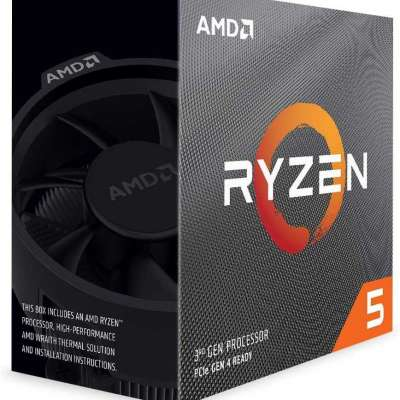Shiv Kandel    added new product for sell.

#### Make home at Chitwan

Rs.8000000 (NPR)

Chitwan, Nepal· In stock· New

In Bharatpur Metropolitan City, 4 kattha of land is available for sale. 1 kattha = Rs. 20 lakhs

For reference
Measurement of area in terms of Bigha

1 bigha (बिघा) = 20 kattha (कठ्ठा) = 6,772.63 m² = 72,900 sq.ft = 13.31 ropani (रोपनी)
1 kattha (कठ्ठा) = 20 dhur (धुर) = 338.63 m² = 3,645 sq.ft.
1 dhur (धुर) = 16.93 m² = 182.25 sq.ft

Measurement of area in terms of Ropani

1 ropani(रोपनी) = 16 aana (आना) = 508.72 m² = 5476 sq. ft

1 aana(आना) = 4 paisa (पैसा) = 31.80 m² = 342.25 sq.ft

1 paisa(पैसा) = 4 daam (दाम) = 7.95 m² = 85.56 sq.ft
1daam (दाम) = 1.99 m² = 21.39 sq.ft
Also,
1 ropani(रोपनी) = 4 matomuri
25 ropani(रोपनी) = 1 khetmuri

### More like thisRs.31000